# Customized Circle packing with R and ggraph

This page follows the previous introduction that explained the basis of circle packing with R and the ggraph library. It describes how to customize color, size, labels and more.

# Bubble size proportionnal to a variable

Mapping the bubble size to a numeric variable allows to add an additionnal layer of information to the chart.

Here, the `vertices` data frame has a `size` column that is used for the bubble size. Basically, it just needs to be passed to the `weight` argument of the `ggraph()` function.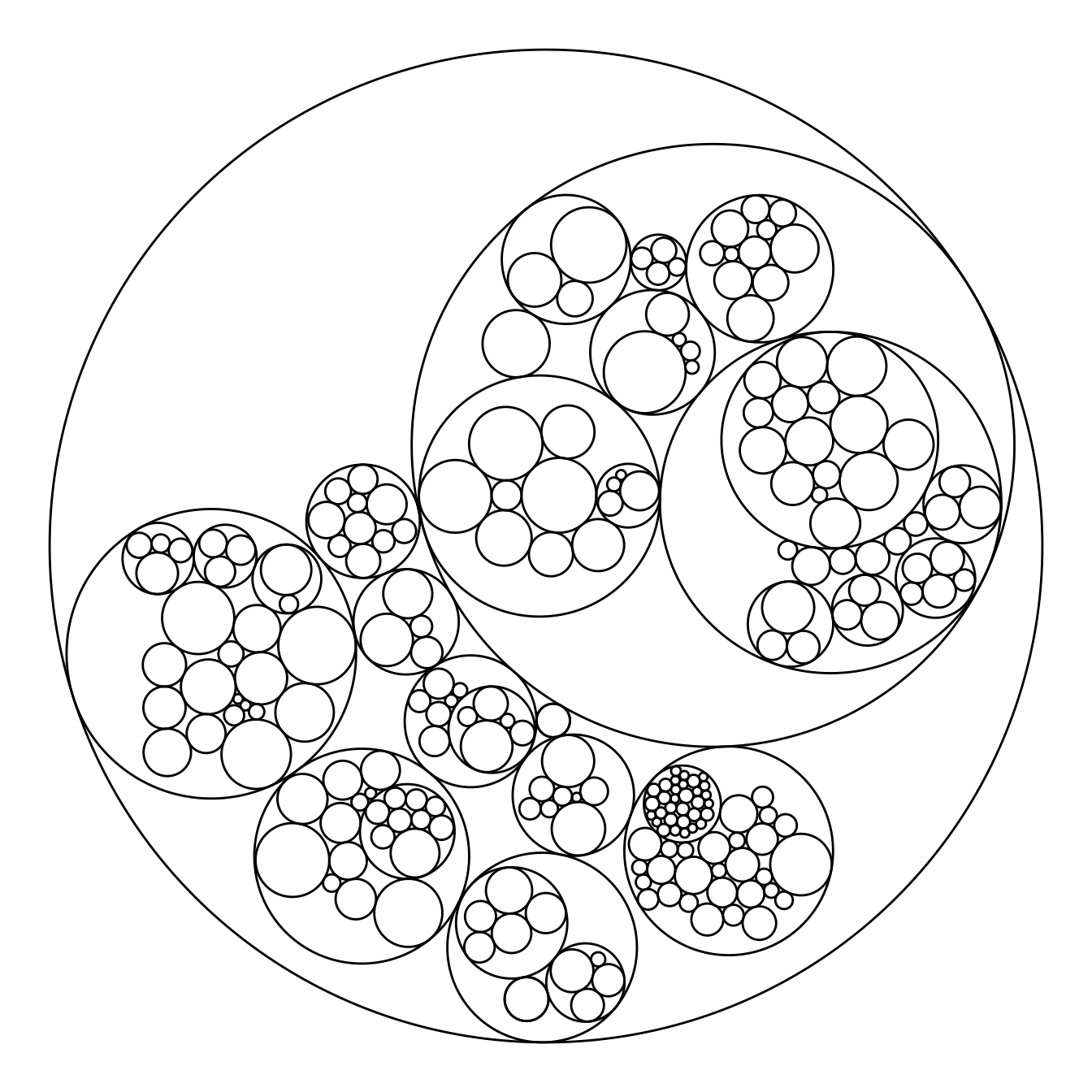``````# Libraries
library(ggraph)
library(igraph)
library(tidyverse)
library(viridis)

# We need a data frame giving a hierarchical structure. Let's consider the flare dataset:
edges <- flare\$edges
vertices <- flare\$vertices
mygraph <- graph_from_data_frame( edges, vertices=vertices )

# Control the size of each circle: (use the size column of the vertices data frame)
ggraph(mygraph, layout = 'circlepack', weight=size) +
geom_node_circle() +
theme_void()``````

# Map color to hierarchy depth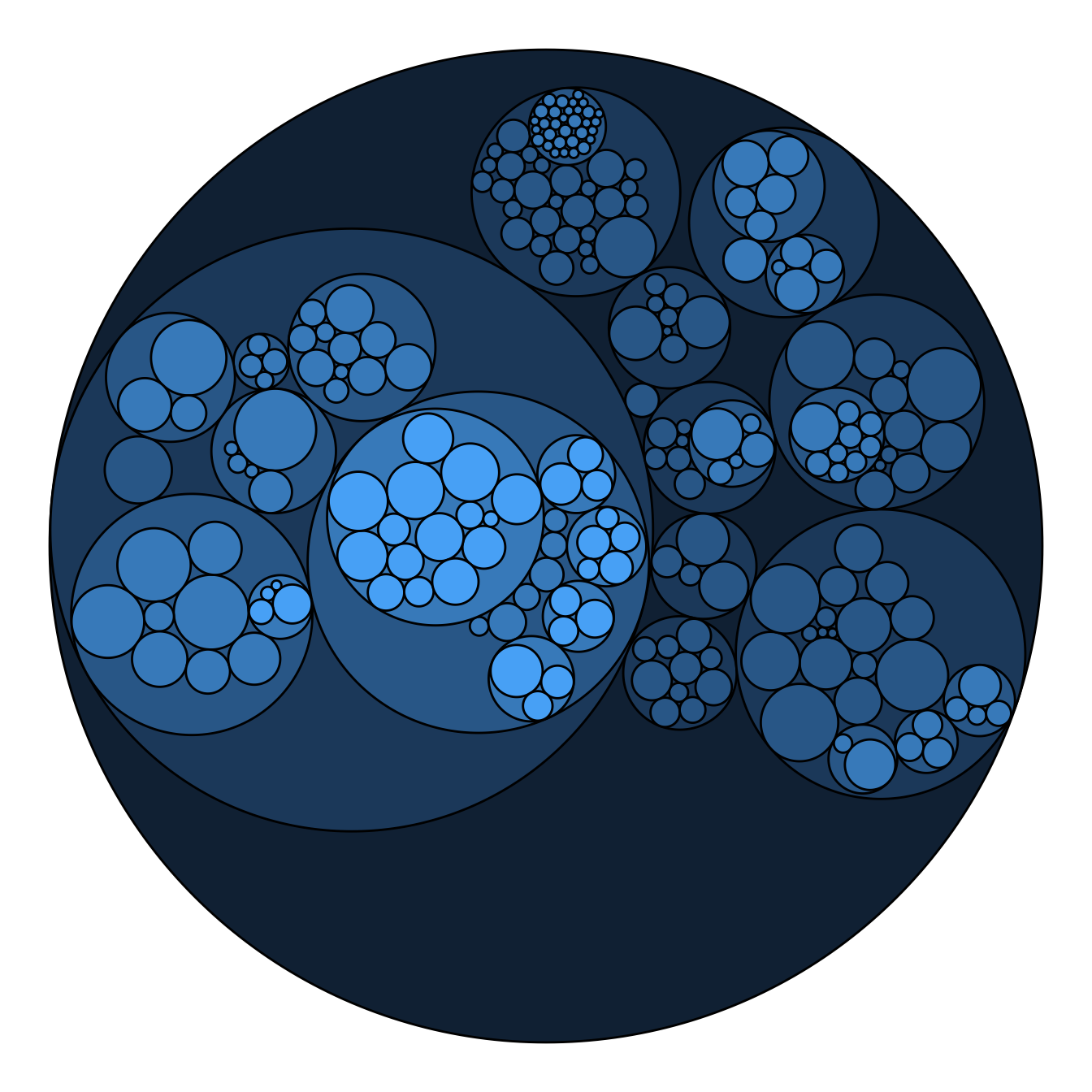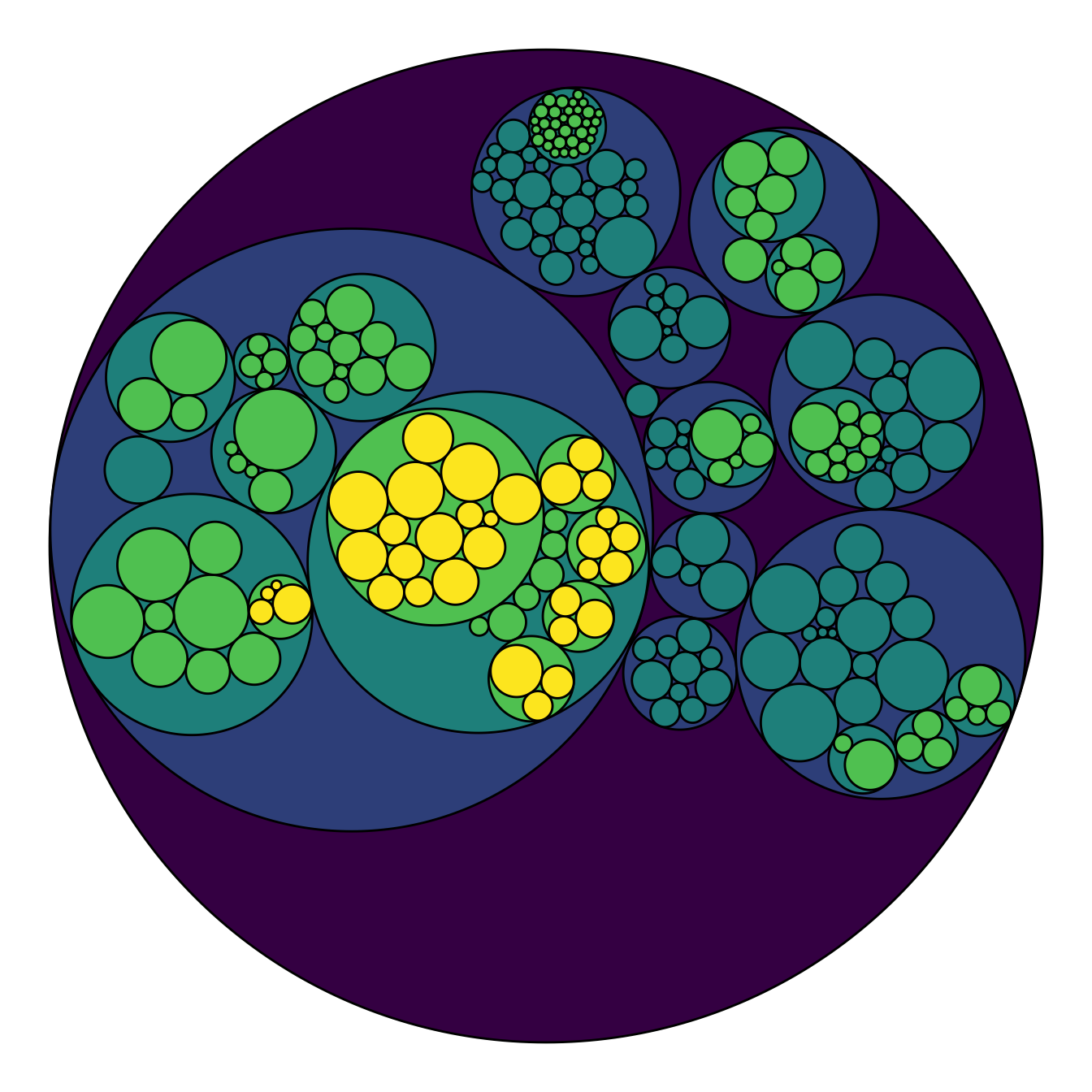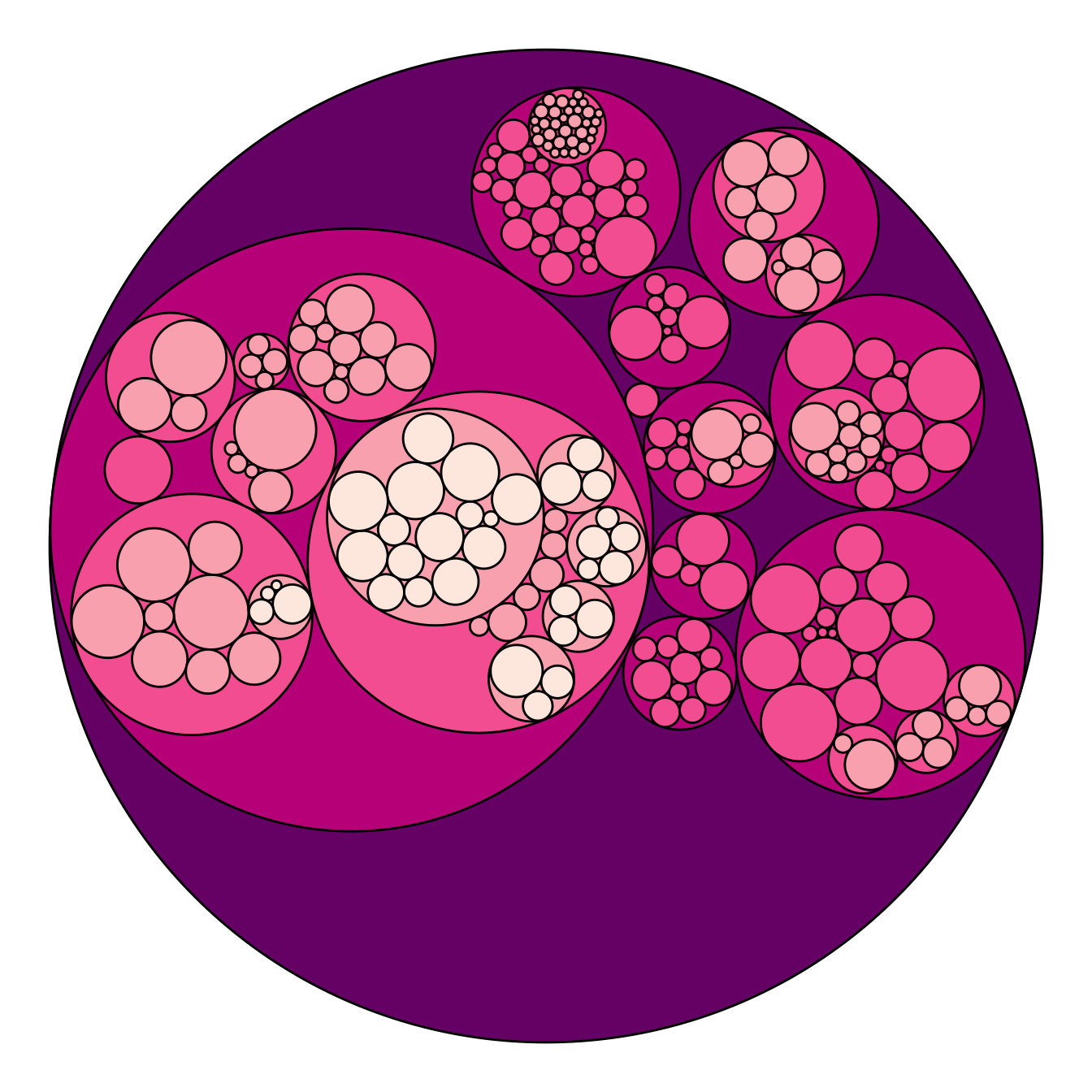Adding color to circular packing definitely makes sense. The first option is to map color to depth: the origin of every node will have a color, the level 1 another one, and so on..

As usual, you can play with the colour palette to fit your needs. Here are 2 examples with the `viridis` and the `RColorBrewer` palettes:

``````# Left: color depends of depth
p <- ggraph(mygraph, layout = 'circlepack', weight=size) +
geom_node_circle(aes(fill = depth)) +
theme_void() +
theme(legend.position="FALSE")
p``````
``````# Adjust color palette: viridis
p + scale_fill_viridis()``````
``````# Adjust color palette: colorBrewer
p + scale_fill_distiller(palette = "RdPu") ``````

# Map color to hierarchy depth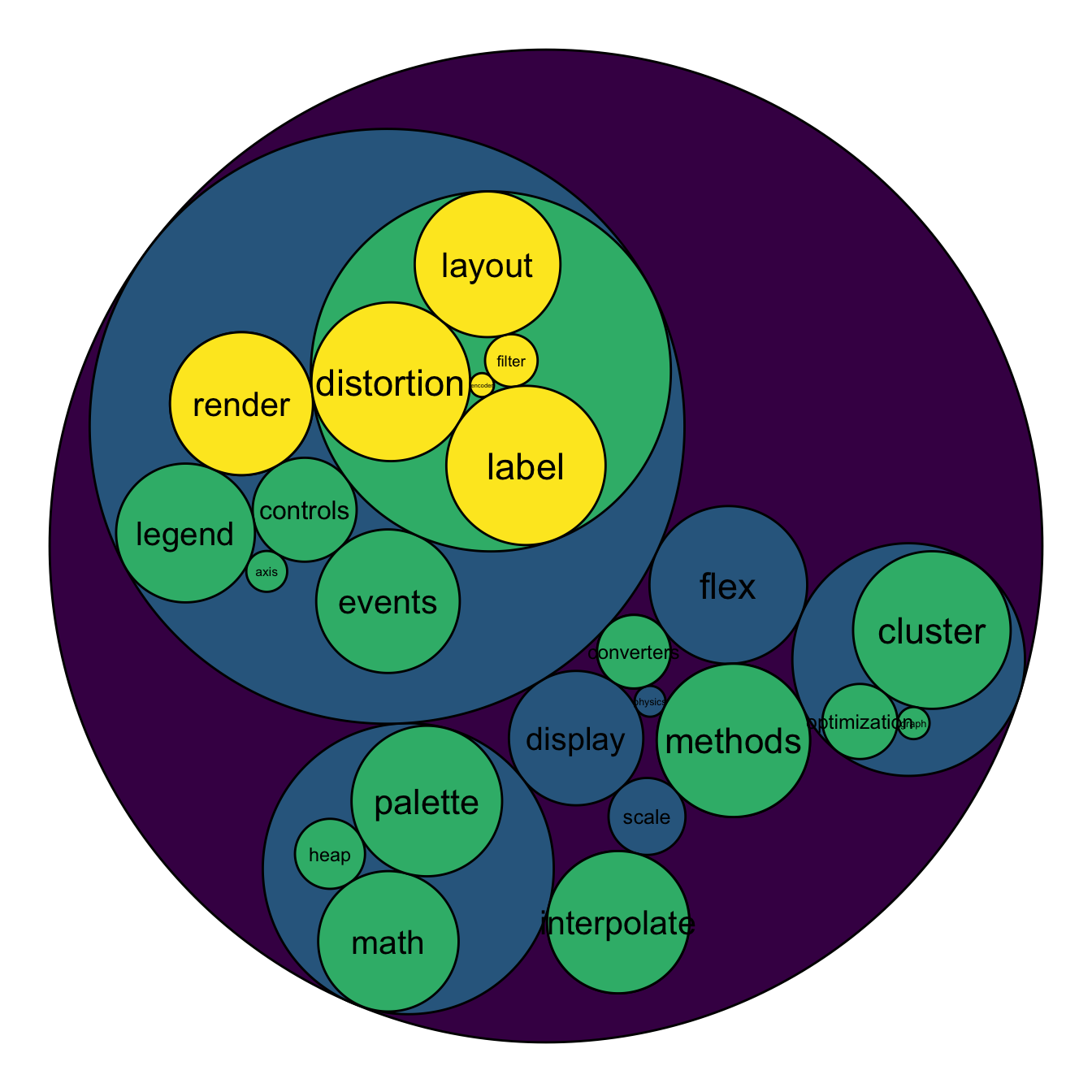To add more insight to the plot, we often need to add labels to the circles. However you can do it only if the number of circle is not to big. Note that you can use `geom_node_text` (left) or `geom_node_label` to annotate leaves of the circle packing:

``````# Create a subset of the dataset (I remove 1 level)
edges <- flare\$edges %>%
filter(to %in% from) %>%
droplevels()
vertices <- flare\$vertices %>%
filter(name %in% c(edges\$from, edges\$to)) %>%
droplevels()
vertices\$size <- runif(nrow(vertices))

# Rebuild the graph object
mygraph <- graph_from_data_frame( edges, vertices=vertices )

# left
ggraph(mygraph, layout = 'circlepack', weight=size ) +
geom_node_circle(aes(fill = depth)) +
geom_node_text( aes(label=shortName, filter=leaf, fill=depth, size=size)) +
theme_void() +
theme(legend.position="FALSE") +
scale_fill_viridis()``````
``````# Right
ggraph(mygraph, layout = 'circlepack', weight=size ) +
geom_node_circle(aes(fill = depth)) +
geom_node_label( aes(label=shortName, filter=leaf, size=size)) +
theme_void() +
theme(legend.position="FALSE") +
scale_fill_viridis()``````

Related chart types

## Contact

This document is a work by Yan Holtz. Any feedback is highly encouraged. You can fill an issue on Github, drop me a message on Twitter, or send an email pasting yan.holtz.data with gmail.com.